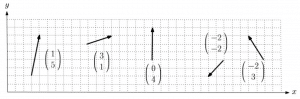# Vectors – quantities with magnitude and direction

## Vectors

Vectors are quantities that have a magnitude and direction. They are typically used to describe the relative position between two points.You may remember first seeing vectors during GCSE Maths when studying translations in shape transformations, for example. You would have also seen them later on in GCSE Maths when studying coordinate geometry. We recap and explore 2D vectors in the first year of A-Level Maths. You will also likely to be expected to use arithmetic and solve problems with them. We explore a brief extension to 3D vectors in the second year of A-Level Maths. See below for the vector topics studied in each year of A-Level Maths.